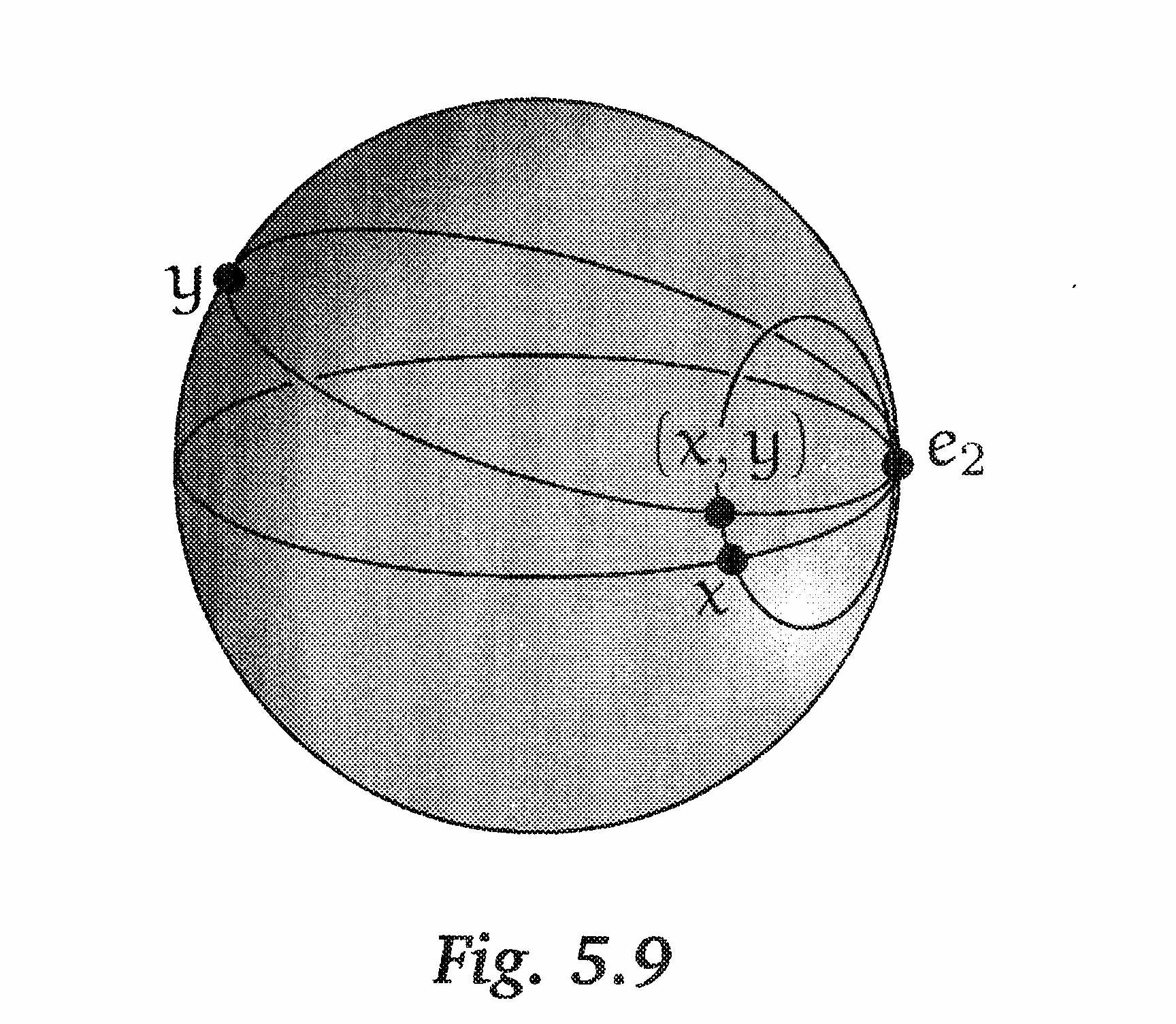# How should I visualize $S^n$ as the reduced suspension of $S^{n-1}$?

Or is there a particularly canonical homeomorphism between $S^n\subset \mathbb{R} ^{n+1}$ and $SS^{n-1}$ (the reduced suspension)? Right now the only thing that comes to mind is to think of the sets $\{t\}\times S^{n-1}, 0 \leq t \leq 1$ in $SS^{n-1}$ as corresponding to the sets $p^{-1}(K)$ where K is a hyperplane in $\mathbb{R}^n$ and $p$ is stereographic projection $S^n\rightarrow \mathbb{R}^n$ from the north pole of $S^n$ (as the $t$ parameter moves from $0$ to $1$ the $K$ sweep out $R^n$ through parallel hyperplanes).

The reduced suspension $S S^{n-1}$ can be represented in $\mathbb{R}^{n+1}$ as the union of two cones on $S^{n-1} \subset \mathbb{R}^{n}\times\{0\},$ one with vertex at $(0,\ldots,0,1),$ and one with vertex at $(0,\ldots,0,-1).$

To get an explicit homeomorphism from this to $S^{n},$ you need not use stereographic projection; instead, just divide every point of $S S^{n-1}$ (each of which is a vector) by its norm.

EDIT: Since you're thinking about the reduced suspension, which I'll call $\Sigma S^{n-1},$ then your identification is probably the best one. You'll end up with a parabolic pencil of $(n-1)$-spheres about the north pole.

• Thank you. I'm a little confused because, if I understand everything correctly, you're talking about the suspension but not the reduced suspension. – Tim kinsella Mar 13 '12 at 19:19
• Oh, gosh, you're right! – Robert Haraway Mar 13 '12 at 19:25

It is convenient to think of this in terms of the smash product $X \# Y$ of two spaces with base point. This is the space $X\times Y$ with $X \vee Y= X \times * \cup * \times Y$ shrunk to a point. Then the reduced suspension of $X$ is really $X \# S^1$. The general result is that

$$S^m \# S^n \cong S^ {m+n}.$$

One way of seeing this is to say that if $S^m$ has the cell structure $e^0 \cup e^m$, and similarly $S^n$, then $S^m \times S^n$ has the cell structure

$$e^0 \cup e^m \cup e^n \cup e^{m+n},$$ and the first three cells determine $S^m \vee S^n$. So when you form the smash product $\ldots$

Here is a figure illustrating $S^1 \# S^1$ from Topology and Groupoids.It is not hard to see that the unreduced suspension is homeomorphic to sphere, so you have to show that the result of collapsing a (standardly embedded) segment in a sphere is still a sphere.

Can you see what the result of collapsing one of the edges of a cube is?

• hmmm. its easy for me to see in low dimensions. I will have to think a bit more. Thank you for your help. – Tim kinsella Mar 13 '12 at 19:29
• Just to clarify: I'm confident I can show that the two spaces are homeomorphic; I was just wondering if there's a particularly natural, standard or enlightening homeomorphism. (Yours is probably all those :) – Tim kinsella Mar 13 '12 at 19:52
• It might help to think of the CW-complex structure, particularly because you can have many different ones! – John Stalfos Apr 12 '12 at 15:09

I think there is a very nice way to see this. But it requires to redefine what the n-sphere should be. So in what follows let $S^n$ denote the one-point compactification of $\mathbb{R}^n$, which is linked to the usual definition via the stereographic projeciton (for example when working in symmetric spectra this approach is very useful since the $\Sigma_n$ just acts by permuting coordinates of $\mathbb{R}^n$ and fixing the point at infinity).

But in this description you can compute explicitely that the identification $\mathbb{R}\times \mathbb{R}^{n-1} \cong \mathbb{R}^n$ induces a homeomorphism $S^1 \wedge S^{n-1} \to S^n$ on the one-point compactifications.